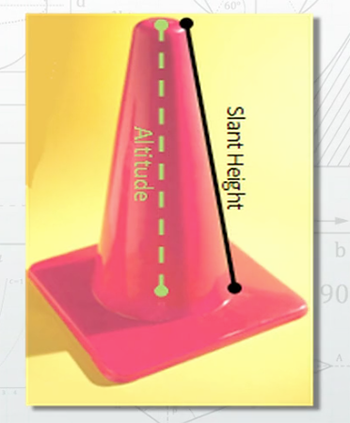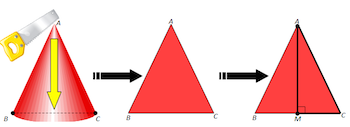# Slant Height: Definition & Formula

An error occurred trying to load this video.

Try refreshing the page, or contact customer support.

Coming up next: How to Multiply and Divide Rational Expressions

### You're on a roll. Keep up the good work!

Replay
Your next lesson will play in 10 seconds
• 0:01 Slant Height vs Altitude
• 1:52 Finding Slant Height
• 3:34 Example
• 5:35 Lesson Summary

Want to watch this again later?

Timeline
Autoplay
Autoplay
Speed

#### Recommended Lessons and Courses for You

Lesson Transcript
Instructor: Miriam Snare

Miriam has taught middle- and high-school math for over 10 years and has a master's degree in Curriculum and Instruction.

This lesson will teach you about the slant height of cones and pyramids. You will learn the formula to calculate the slant height. After the lesson, you will be able to test your knowledge with a quiz.

## Slant Height vs. Altitude

Figures such as cones and pyramids have two measurements that indicate how tall the figure is. One of these measurements is called the slant height and the other is called the altitude.

Let's consider a traffic cone to help us learn the difference between these two measurements. If you measure the distance along the outside of the cone from the top to the base, that measurement is the slant height. To measure it, you have to place the yardstick at a slant.

If you drop a yardstick straight down through the hole in the top of the cone until it hits the bottom, that measurement is the altitude. The yardstick in this case will make a right angle at the center of the base. Take a look at the picture to see these measurements labeled.Note that the dashed segment of the altitude indicates that it is inside of the cone.

Our traffic cone is a little different from the geometric shape called a cone. In geometry, the base of a cone is only a circle that does not extend beyond the opening of the cone. The point of a cone in geometry is called the vertex point. The slant height and the altitude always meet at that vertex point in a cone. On the traffic cone, the two segments did not meet because the tip is flat and does not come to one point.

In a pyramid, you also have a slant height and an altitude. Imagine you are standing at the top of a pyramid at the vertex point, and you want to get back to ground level. You have two options. You can slide down the center of the triangular side of the pyramid - the slant height - to the outside of the pyramid. Otherwise, you can drop straight down through a hole in the top and land in the middle of the inside of the pyramid - the altitude.

## Finding Slant HeightTo calculate the slant height of either a cone or a pyramid, you need to imagine that you can look inside of the figure. Let's cut open a cone as an example. First, we cut down through the cone from vertex point A to segment BC to get two halves. The cut surface of either half is now in the shape of an isosceles triangle, which is a triangle with two sides that are the same length. Those two sides were the slant height of the cone. We now have triangle ABC, where sides AB and AC have the same length.

Now, we draw in the altitude of the cone from A straight down to BC, so that a right angle is created. Point M is the point where the altitude meets BC. Triangle AMC is a right triangle where AM is the altitude and AC is the slant height of the original cone. AC is also the hypotenuse of the right triangle, since it is the side opposite the right angle.

Every cone and pyramid contains a right triangle if we cut up the figure like we did in this example.

We can use the Pythagorean theorem, a^2 + b^2 = c^2, to calculate the slant height. For both cones and pyramids, a will be the length of the altitude and c will be the slant height. For a cone, b is the radius of the circle that forms the base. The radius is the distance from the center of the base to the edge of the base. For a pyramid, b is half the length of the side of the square that forms the base.

## Finding Slant Height - Example

Let's go through an example where we calculate the length of the slant height of a pyramid. In this example, we are given that the altitude of the pyramid measures 8 inches and each side of the base is 12 inches long. The altitude is the dashed red segment DM and the slant height is the purple segment DY.

To unlock this lesson you must be a Study.com Member.

### Register to view this lesson

Are you a student or a teacher?

### Unlock Your Education

#### See for yourself why 30 million people use Study.com

##### Become a Study.com member and start learning now.
Back
What teachers are saying about Study.com

### Earning College Credit

Did you know… We have over 160 college courses that prepare you to earn credit by exam that is accepted by over 1,500 colleges and universities. You can test out of the first two years of college and save thousands off your degree. Anyone can earn credit-by-exam regardless of age or education level.